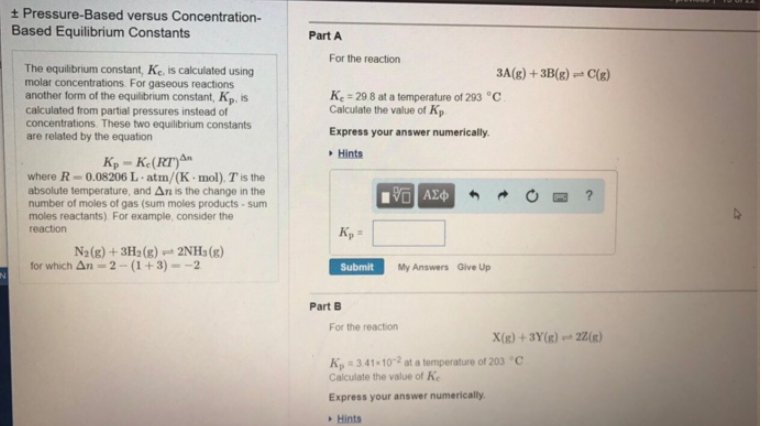Chemistry Practice Problems Equilibrium Expressions Practice Problems Solution: The equilibrium constant, Kc, is calculated using ...

# Solution: The equilibrium constant, Kc, is calculated using molar concentrations. For gaseous reactions another form of the equilibrium constant, Kp, is calculated from partial pressures instead of concentrations. These two equilibrium constants are related by the equation Kp = Kc (RT)Δn where R = 0.08206 L•atm/(K•mol), T is the absolute temperature, and Δn is the change in the number of moles of gas (sum moles product - sum moles reactants). For example, consider the reaction N2(g) + 3H2(g) ⇌ 2NH3(g)  for which Δn = 2 - (1 + 3) = -2 Part A. For the reaction 3A(g) + 3B(g) ⇌ C(g) Kc = 29.8 at a temperature of 293°C. Calculate the value of Kp. Express your answer numerically. Part B. For the reaction X(g) + 3Y(g) ⇌ 2Z(g) Kp = 3.41 x 10-2 at a temperature of 203°C. Calculate the value of Kc. Express your answer numerically.

###### Problem

The equilibrium constant, Kc, is calculated using molar concentrations. For gaseous reactions another form of the equilibrium constant, Kp, is calculated from partial pressures instead of concentrations. These two equilibrium constants are related by the equation

Kp = Kc (RT)Δn

where R = 0.08206 L•atm/(K•mol), T is the absolute temperature, and Δn is the change in the number of moles of gas (sum moles product - sum moles reactants). For example, consider the reaction

N2(g) + 3H2(g) ⇌ 2NH3(g)

for which Δn = 2 - (1 + 3) = -2

Part A.

For the reaction

3A(g) + 3B(g) ⇌ C(g)

Kc = 29.8 at a temperature of 293°C. Calculate the value of Kp. Express your answer numerically.

Part B.

For the reaction

X(g) + 3Y(g) ⇌ 2Z(g)

Kp = 3.41 x 10-2 at a temperature of 203°C. Calculate the value of Kc. Express your answer numerically.View Complete Written Solution

Equilibrium Expressions

Equilibrium Expressions

#### Q. Part 1 (1 point) Do not add states of matter to the equilibrium expressions.Write the equilibrium constant expression for the following reaction: N2O ...

Solved • Thu Oct 04 2018 13:18:30 GMT-0400 (EDT)

Equilibrium Expressions

#### Q. At 2000 K the partial pressures of an equilibrium mixture of H2S, H2, and S are 0.015, 0.041, and 0.025 atm, respectively. Calculate the value of the ...

Solved • Tue Sep 18 2018 16:33:17 GMT-0400 (EDT)

Equilibrium Expressions

#### Q. Calculate the value of the equilibrium constant K for the reaction 2D ⇌ A + 2B from the following information A + 2B ⇌ C             Kc = 3.15C ⇌ 2D  ...

Solved • Tue Sep 18 2018 13:28:46 GMT-0400 (EDT)

Equilibrium Expressions

#### Q. The reaction 2PH3 (g) + As2 (g) ⇌ 2AsH3 (g) + P2 (g) has Kp = 2.9 x 10-5 at 873 K. At the same temperature, what is Kp for each of the following react...

Solved • Mon Sep 17 2018 19:25:57 GMT-0400 (EDT)